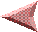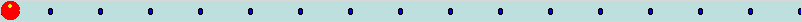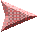PreviousA game using variables and decisionsNext

Every piece of memory in a computer has a location. The very first piece is at location 0, the next is at location 1, and so forth.

Early computer programmers had to keep track of all the locations and write programs like

`add the contents of address 12345 to the contents of address 54321`.

Like so many things in the bad old days, this got fixed. In modern programming languages we give important places in memory a name, and then we write programs like

```Add the contents of the memory named number_of_dogs to the contents of the memory named number_of_cats```.

We call `number_of_dogs` and `number_of_cats` variables, because the value saved in that memory location can vary.

In Tcl/Tk, we shorten the string `the contents of memory named` to a dollar sign, so we'd write something like

`add \$number_of_dogs to \$number_of_cats`.

At some point we've got to tell the computer how many dogs and cats we have.

The command that will assign a value to a variable is `set`.

The `set` command uses two arguments. The first is the name of a variable, and the second is the value to put in that variable.

To assign the value `1` to the variable `number_of_dogs` we'd use code like this:

 ``` set number_of_dogs 1 ```

Now that we can assign a number to a variable, we'll want to do things with the numbers.

For instance, we might want to know haw many animals there are if we have `\$number_of_dogs` and `\$number_of_cats`.

To do arithmetic in Tcl/Tk, we need another new command.

The command Tcl/Tk uses to do arithmetic is named `expr`. The `expr` command understands simple algebra and does calculations. The `expr` command can do lots of things with numbers, and we'll talk about more of them later. Here's a few of the things it understands:

Symbol Example Description
`+` `\$a + \$b` Add two numbers
`-` `\$a - \$b` Subtract the second number from the first
`*` `\$a * \$b` Multiply two numbers
`/` `\$a / \$b` Divide the first number by the second
`%` `\$a % \$b` Divide the first number by the second and return the remainder

So, to calculate the total number of pets using Tcl/Tk we'd really write this:

 ``````set number_of_dogs 1 set number_of_cats 2 expr \$number_of_dogs + \$number_of_cats ``````

This `expr` command calculates how many pets we own, but doesn't do anything with the total.

What we probably want to do is save the result.

Now for a tricky bit.

Most of the commands in Tcl/Tk not only do something (like add two numbers), but they also return a value. That value isn't in a variable, it's just there. You can do stuff with it. Like, you can assign it to a variable.

In Tcl/Tk, we use square brackets to tell Tcl that we want to grab the return value from a command and do something with it.

To assign the results of using `expr` to count how many pets we have, we'd write something like this:

 ``````set number_of_dogs 1 set number_of_cats 2 set number_of_pets [expr \$number_of_dogs + \$number_of_cats] ``````

That says to make the number of dogs 1, the number of cats 2, and then add the two together and assign the sum to the variable `number_of_pets`. The square brackets tell Tcl/Tk to take the value that the `expr` command returns and do stuff with it.

In this case, the stuff we'll do with the return is to treat the return value as the second argument to the set command.

You can think of it as Tcl/Tk running the command inside of the square brackets and then replacing everything that was inside the brackets with whatever that code returned.

The Tcl/Tk interpreter goes through 3 steps to count how many pets we own. First, it looks at the original Tcl/Tk code we wrote. That looks like this:

 ``````set number_of_dogs 1 set number_of_cats 2 set number_of_pets [expr \$number_of_cats + \$number_of_dogs] ``````

Next the interpreter finds all the variables, and replaces the dollarsign-variable-name strings with the value that's stored in that variable.

After this step, the code looks like this:

 ``````set number_of_dogs 1 set number_of_cats 2 set number_of_pets [expr 2 + 1] ``````

The next step is for Tcl/Tk to find all the square braces, look at the commands inside the square braces, and replace that string with the return value of the commands in the square braces.

After this step, the Tcl/Tk interpreter is looking at commands like this:

 ``````set number_of_dogs 1 set number_of_cats 2 set number_of_pets 3 ``````

So, if you have 10 pounds of pet food, and each dog eats 1/2 pound of food per day, and each cat eats 1/4 pound of food per day, can you calculate how long it takes the train to go from Boston to New York?

OK, maybe that's not the right information to calculate a train trip.

But, you can calculate how long the food will last.

Each day, the pets will eat this much food:

 `(\$number_of_dogs * .5) + (\$number_of_cats * .25)`

If you divide the total amount of food by the amount the pets eat each day, that will be the number of days the food will last.

You can write that like this:

 ``````set number_of_dogs 1 set number_of_cats 2 set pounds_of_food 10 set food_per_day [expr (\$number_of_dogs * .5) + (\$number_of_cats * .25)] set number_of_days [expr \$pounds_of_food / \$food_per_day] tk_messageBox -type ok -message "You've got \$number_of_days days of food" ``````

Take a break now and start up Komodo Edit. Try writing some short programs to count how many pets you have and how long the food will last. Add in some gerbils that eat 1/8 (.125) pounds of food per day.

Let's get back to important stuff like writing computer games...

The previous number guessing game was pretty lame. The right answer was always the same. When we write computer games, we want to make the computer do different things. We want it to have different secret numbers each time we play the game, or get a new set of cards each time we play solitaire.

We call these different things random events.

Since the computers use numbers for everything, we create a random event as a number and then decide what each number means.

Most computer languages have a random number generator in them. The common random number generator gives you a fraction between 0 and .99999.

The reason for using a fraction between 0 and .99999 is that we can easily convert that fraction to a number in whatever range we need it to be. It doesn't matter what range of values the guy who wrote the random number generator used, people will always need something different. Starting with a fraction is the simplest way to do the calculations.

If you need a number between 0 and 10, you just multiply the random number by 10 which will give you a number between 0 and 9.999.

For example, Here's how to get a random number between 1 and 6, as if you just rolled a 6 sided dice: you multiply the randomly chosen fraction by 6 to convert the fraction to a number between 0 and 5.99999.

Nobody has a set of dice that roll fractions like 5.999, so there's another command that will take a number with a fraction, strip off the fraction part and return the whole number. That will give you a random number between 0 and 5.

Nobody has dice that go from 0 to 5, so we add 1, and that makes a random number between 1 and 6.

In Tcl, the random number generator and the command to get rid of the fraction are part of the `expr` command. You can use these commands as part of an arithmetic expression. You can even group the parts of the arithmetic command with parentheses like you would in algebra.

The two `expr` commands for making random numbers are

 `rand()` Return a random fraction between 0 and .9999 `int(value)` Strip off any fraction and return only the integer part of a value.

You might notice the parentheses in the `rand` and `int` commands. That's because these are arithmetic functions, like sin and cosine. If you haven't studied trigonometry yet, don't worry, just put in the parentheses and everybody will be happy.

The final trick in converting the previous game so that it will choose a different secret number each time is for the computer to compare the value associated with the button to the secret and decide whether that's a win or lose.

The Tcl/Tk command for doing a comparison is the `if` command. You tell the `if` command what test to run, and what to do if the test succeeds or fails.

The test is a question that can be answered as TRUE or FALSE. It's usually a comparison. Tcl/Tk uses arithmetic symbols to do these comparisons. Here's a few of them

Symbol Definition Example Description
`==` Tests for equality. `\$first == \$second` Is the value in the variable named first the same as the value in the variable named second
`!=` Tests for inequality. `\$first != \$second` Is the value in the variable named first is NOT the same as the value in the variable named second
`<` Tests for less than `\$first < \$second` Is the value in the variable named first less than the value in the variable named second
`>` Tests for greater than `\$first > \$second` Is the value in the variable named first greater than the value in the variable named second
`<=` Tests for less than or equal to `\$first <= \$second` Is the value in the variable named first less than or equal to the value in the variable named second
`>=` Tests for greater than or equal to `\$first >= \$second` Is the value in the variable named first greater or equal to than the value in the variable named second

The definition of the command looks like this:

 Syntax:` if {test} {action} ` evaluates the test. If the test evaluates as TRUE the script `action` is evaluated.

We commonly write the `if` command on three or more lines. The first line has the if command and the test, and ends with an open curly bracket. The next lines have the commands to do if the test is TRUE, and the last line is a close curly bracket to match the one on the first line.

Look at the curly brackets in the `if` command in the example below. The space between the `if` and the first curly brace needs to be there, as does the space between the closing curly brace in the test and the opening curly brace for the command.

The number sign (#) is called a comment character. It means that everything on this line is for people, not computers. The Tcl/Tk interpreter will ignore that line. Comments are a good way to tell people what the program is supposed to be doing.

The other reason for comments is that it helps you remember what you meant when you wrote some code and need to use it later. You think you'll never forget what something means when you are writing it, but even a day later it won't make sense unless you put in some comments.

 `````` # Show two labels to explain the game. label .info1 -text "I've got a secret number." label .info2 -text "Can you guess it?" grid .info1 .info2 # Calculate a secret number between 1 and 2 set secret [expr 1 + int(rand() * 2)] # If the secret is 1, make .one the winning button if {\$secret == 1} { button .one -text "1" -command {tk_messageBox -type ok -message "You Win"} button .two -text "2" -command {tk_messageBox -type ok -message "You Lose"} } # If the secret is 2 , make .two the winning button if {\$secret == 2} { button .one -text "1" -command {tk_messageBox -type ok -message "You Lose"} button .two -text "2" -command {tk_messageBox -type ok -message "You Win"} } grid .one .two ``````

Type (or copy/paste) this game into Komodo Edit and play with it a little bit. Try changing the order of the widgets (`.info1`, `.info2`, `.one` and `.two` in the grid command. Then try changing the messages in the `tk_messageBox` commands.

This lesson introduced several new ideas and commands. The important points in this lesson are:

• A variable is a place in memory where the computer stores information. The Tcl/Tk interpreter lets you reference that location with a name that you make up.

• Your program assigns a value to a variable with the `set` command.

• Your program can do arithmetic with the `expr` command.

• The `expr` command can generate a random number between 0 and .9999 with the `rand()` function.

• Your program can convert a number between 0 and .999 to an integer by multiplying and using the `int()` function.

• Your program can compare the value of one variable with another using the `if` command.

This game is less lame than it was. We still have a ways to go before the games get really interesting, though.

For instance, if you were making a game to guess a number between 1 and 100, you wouldn't want to type 100 different `if` commands with 100 lines of `button` commands in each one.

Why not have the computer do all the work? We'll look at loops in the next lesson.PreviousNext